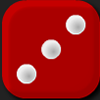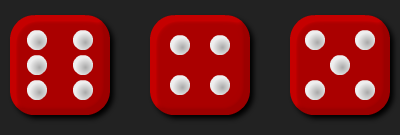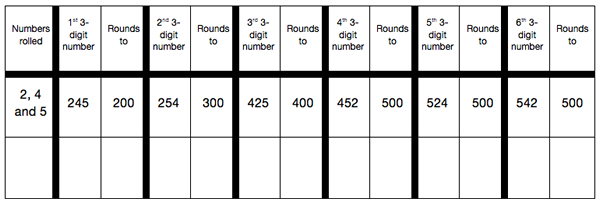#### You may also like### Round the Four Dice

This activity involves rounding four-digit numbers to the nearest thousand.### Round the Dice Decimals 2

What happens when you round these numbers to the nearest whole number?### Round the Dice Decimals 1

Use two dice to generate two numbers with one decimal place. What happens when you round these numbers to the nearest whole number?

# Round the Three Dice

## Round the Three DiceThere are three dice, each of them with faces labelled from 1 to 6.
When the dice are rolled they can be combined in six different ways to make a three-digit number.

For example, if I roll a 2 and a 4 and a 5, I can combine them to make 245, 254, 425, 452, 524 or 542.

Now round each of these numbers to the nearest 100:
245 rounds to 200, 254 rounds to 300, 425 rounds to 400, 452 rounds to 500, 524 rounds to 500 and 542 rounds to 500.

Repeat for other rolls of the dice.

Can each of the six numbers round to the same multiple of 100?
Can each of the six numbers round to a different multiple of 100?

There some interactive dice here that you can use for this problem.

#### Why do this activity?

This activity provides a meaningful task for practising rounding three-digit numbers to the nearest multiple of 100. It encourages children to record their results, notice patterns and make predictions.

#### Possible approach

A possible starting point is using a number line to remind the class what is meant by rounding.
The interactive dice can be used to model the activity on an interactive whiteboard or can be used by the children as they engage with the task. You may also find that individual dice are useful.

As the task is being modelled, the results should be recorded in a table, perhaps like the one below:This organisation of results will support the children to notice patterns and conjecture about when numbers will round to different multiples of 100.

Some children may move onto the extension tasks (below).

#### Key questions

Which numbers can we make?
What will they round to? Will they round up or down? Why?
Do they round to the same multiple of 100? Why? When will this happen?
Does it make a difference if the digits rolled are unique?

#### Possible extensions

Extension 1: Did the class find examples where some of the three-digit numbers round to the same multiple of 100? Can they then come up with a rule about when this will happen? When will two round to the same? Or three? Or four? Or five? Or all six?
Extension 2: Did the class find examples where each of the three-digit numbers round to a different multiple of 100? Why? Why not?
Extension 3: Having completed the original task, ask the children to add a column to the right hand side of their table to note when numbers round up or down. Can they predict from the initial dice roll, how many of the three-digit numbers made will round up or down?
Extension 4: What if you change the numbers on the faces of the dice? Can you pick numbers so that all of the six three-digit numbers round to the same multiple of 100? Can you pick numbers so that all of the six three-digit numbers round to a different multiple of 100?
Extension 5: What happens if you round to the nearest multiple of 10 instead of the nearest multiple of 100?
Extension 6: Have a go at the activity Round the Four Dice and practise rounding numbers to the nearest multiple of 1000.

#### Possible support

Copies of the table to write straight into would be handy.
A number line might also be useful to support children in reasoning about which multiple of 100 is closest to a given number.
Children may like to try Round the Two Dice before tackling this task.